# Multifield validation

Tags

Multiple choice

What will this output? The URL is something like: `...something.php?dish=Corndog`

1. <?php
2. \$dish = \$_GET['dish'];
3. \$isYummy = 'yes';
4. if (\$dish == 'corndog') {
5.     \$isYummy = 'no';
6. }
7. print \$isYummy;A

yes

B

no

C

Can't tell from the information given.

Not graded. So why do it?

Let's extend what we just did. Let's put two or more values at the end of the URL.

### The goal

Let's pass in a price, as well as the state. Try this:

https://webappexamples.skilling.us/validation/basics/multifield-sales-tax/multifield-sales-tax.php?state=mi&price=100

The GET params are `?state=mi&price=100`.

Here's what you should see:What if there's bad data? Here's what you should see if both data fields are missing: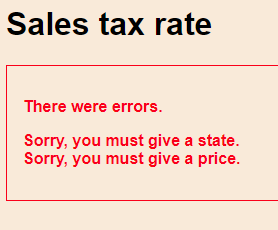What if both values are there, but invalid?

https://webappexamples.skilling.us/validation/basics/multifield-sales-tax/multifield-sales-tax.php?state=mssi&price=something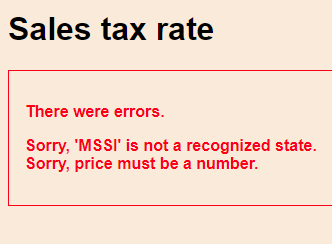Or maybe just one is invalid.

https://webappexamples.skilling.us/validation/basics/multifield-sales-tax/multifield-sales-tax.php?state=mi&price=-100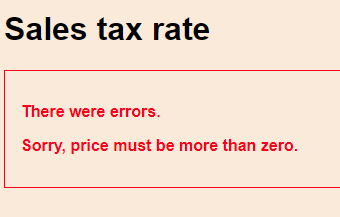We want code that shows all errors that apply. It only shows results when there are no errors at all.

You can dowload the code.

### Validation

The code is mostly the same, except:

• One error message variable for each input
• After each field is checked, combine the error messages into one

Here's what the code will do: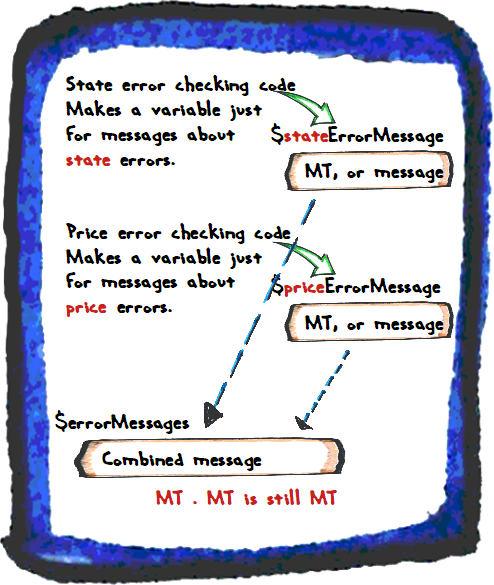Each input has its own error message. After all the checks are done, we'll combine all of the error messages. If all of the error message variables are MT, then their combination will be MT as well. We can use that as a flag.

Start like this:

• // Set up vars to track results.
• \$errorMessages = '';
• \$taxRate = 0;
• \$stateShortName = '';
• \$stateLongName = '';
• \$price = 0;
• \$tax = 0;
• \$total = 0;

A new variable, `\$errorMessages` (note the plural) will be used later to combine the errors for each field.

Now lets check the state. Almost the same as before, but with a variable that's for tracking state errors only.

• // State validation.
• \$stateErrorMessage = '';
• // Is there input?
• if (!isset(\$_GET['state'])) {
•     \$stateErrorMessage = 'Sorry, you must give a state. <br> ';
• }
• if (\$stateErrorMessage == '') {
•     // There is a state.
•     \$stateShortName = strtoupper(trim(\$_GET['state']));
•     // Is the state known?
•     if (\$stateShortName != 'MI' && \$stateShortName != 'IL') {
•         \$stateErrorMessage = "Sorry, '\$stateShortName' is not a recognized state.<br>";
•     }
• }

The state has its own error message variable. Also, we've added a `br` tag to the end of the error message values, since more than one error message can be shown. The `br` will make sure that each message is on its own line. You'll see later how that makes sense.

Now for the price.

• // Price.
• \$priceErrorMessage = '';
• if (!isset(\$_GET['price'])) {
•     \$priceErrorMessage = 'Sorry, you must give a price.<br>';
• }
• // Numeric check.
• if (\$priceErrorMessage == '') {
•     // Get the price.
•     \$price = \$_GET['price'];
•     // Check that price is numeric.
•     if (!is_numeric(\$price)) {
•         \$priceErrorMessage = 'Sorry, price must be a number.<br>';
•     }
• }
• // Range check.
• if (\$priceErrorMessage == '') {
•     if (\$price <= 0) {
•         \$priceErrorMessage = 'Sorry, price must be more than zero.<br>';
•     }
•     if (\$price >= 1000000) {
•         \$priceErrorMessage = 'Sorry, price is too high to be real.<br>';
•     }
• }

First, make a variable for error messages about the price. Check if the price was given at the end of the URL:

• if (!isset(\$_GET['price'])) {
•     \$priceErrorMessage = 'Sorry, you must give a price.<br>';
• }

Now, check whether the value given is a number. Only run the check if we know there have been no price errors so far.

• // Numeric check.
• if (\$priceErrorMessage == '') {
•     // Get the price.
•     \$price = \$_GET['price'];
•     // Check that price is numeric.
•     if (!is_numeric(\$price)) {
•         \$priceErrorMessage = 'Sorry, price must be a number.<br>';
•     }
• }

`!is_numeric(\$price)` is the PHP for `Not IsNumeric(sPrice)` in VBA.

Next check. Even if a price is given, and it is a number, it might be a silly number, like -3123, or 1939293999393. We'll add a range check.

• // Range check.
• if (\$priceErrorMessage == '') {
•     if (\$price <= 0) {
•         \$priceErrorMessage = 'Sorry, price must be more than zero.<br>';
•     }
•     if (\$price >= 1000000) {
•         \$priceErrorMessage = 'Sorry, price is too high to be real.<br>';
•     }
• }

As before, only do the check if there have been no other price errors.

OK. We've checked both fields. Now, let's combine the error messages into one:

• // Combine the error messages.
• \$errorMessages = \$stateErrorMessage . \$priceErrorMessage;

VBA's `&` operator, for appending strings together, is `.` in PHP.

Reflect

If there are no errors, what will `\$errorMessages` be after the line above runs?

If you were logged in as a student, the lesson would pause here, and you'd be asked to type in a response. If you want to try that out, ask for an account on this site.Georgina

Well, `\$stateErrorMessage` will be MT, and `\$priceErrorMessage` will be MT, too.

Append MT to MT..., I'd guess that gives you MT. Like 0 + 0 = 0.

Right! Good work.

Here's that line again:

• // Combine the error messages.
• \$errorMessages = \$stateErrorMessage . \$priceErrorMessage;

We could have two error messages. We want to put them on separate lines, like this:That's why we put a `br` tag at the end of the messages, like this:

• \$stateErrorMessage = "Sorry, '\$stateShortName' is not a recognized state. <br> ";

To make sure that when the messages were put together, they'd be displayed on separate lines.

Now we can add a check to decide whether to do any processing.

• // Should there be processing?
• if (\$errorMessages == '') {
•     // No input errors.
•     if (\$stateShortName == 'MI') {
•         \$stateLongName = 'Michigan';
•         \$taxRate = 0.06;
•     }
•     else {
•         \$stateLongName = 'Illinois';
•         \$taxRate = 0.0625;
•     }
•     \$tax = \$price * \$taxRate;
•     \$total = \$price + \$tax;
• }
• ?><!doctype html>

That's the end of the top chunk. Everything's ready for output.

### The bottom chunk

For the bottom chunk, we might add some styling, and put the error messages in container.

• <h1>Something</h1>
• <?php
• if (\$errorMessages != '') {
•     print "<div class='error-message'>
•              <p>There were errors.</p>
•              <p>\$errorMessages</p>
•            </div>\n";
• }
• else {
•     // Output stuff here.
• }
• ...
• .error-message {
•     color: red;
•     font-weight: bold;
•     border: red solid thin;
•     margin: 1rem;
• }

A nice thing about this pattern, is that it works, no matter how many inputs there are.

Four inputs?

• Make an error message variable for each field.
• Combine them into one composite error message.
• Processing only if the composite message is MT.

Ten inputs?

• Make an error message variable for each field.
• Combine them into one composite error message.
• Processing only if the composite message is MT.

The same thing.

### Yet another example

Let's write a program that reports the results of an MSU vs UM football game.

With a URL like `...football-scores.php?msu=33&um=5`, you'll get: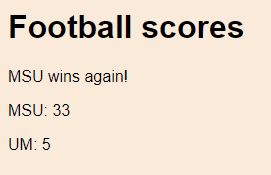https://webappexamples.skilling.us/validation/multifield/football-scores/football-scores.php

https://webappexamples.skilling.us/validation/multifield/football-scores/football-scores.php?msu=33

https://webappexamples.skilling.us/validation/multifield/football-scores/football-scores.php?um=failwhale

https://webappexamples.skilling.us/validation/multifield/football-scores/football-scores.php?msu=33&um=5

https://webappexamples.skilling.us/validation/multifield/football-scores/football-scores.php?msu=20&um=25

Here's how the code will work.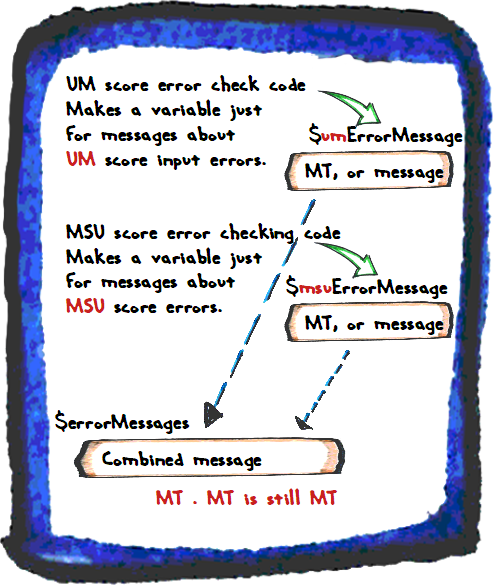Let's compare the code for the state sales tax thing, with this one. Here's the code that starts them both:

• <?php
• // Set up vars to track results.
• \$errorMessages = '';
• \$taxRate = 0;
• \$stateShortName = '';
• \$stateLongName = '';
• \$price = 0;
• \$tax = 0;
• \$total = 0;
•
•
• <?php
• // Set up vars to track results.
• \$errorMessages = '';
• \$umScore = 0;
• \$msuScore = 0;

Both programs initialize variables. They have the variable `\$errorMessages` in common, but all the other variables depends on the task.

Next, there's the validation of one of the inputs.

1. // State validation.
2. \$stateErrorMessage = '';
3. // Is there input?
4. if (!isset(\$_GET['state'])) {
5.     \$stateErrorMessage = 'Sorry, you must give a state.<br>';
6. }
7. if (\$stateErrorMessage == '') {
8.     // There is a state.
9.     \$stateShortName = strtoupper(trim(\$_GET['state']));
10.     // Is the state known?
11.     if (\$stateShortName != 'MI' && \$stateShortName != 'IL') {
12.         \$stateErrorMessage = "Sorry, '\$stateShortName' is not a recognized state.<br>";
13.     }
14. }
15.
16.
17. // UM score validation.
18. \$umErrorMessage = '';
19. // Value given?
20. if (!isset(\$_GET['um'])) {
21.     \$umErrorMessage = 'Sorry, you must give a score for UM.<br>';
22. }
23. // Numeric check.
24. if (\$umErrorMessage == '') {
25.     // Get the score.
26.     \$um = \$_GET['um'];
27.     // Check score is numeric.
28.     if (!is_numeric(\$um)) {
29.         \$umErrorMessage = 'Sorry, the UM score must be a number, like 0.<br>';
30.     }
31. }
32. // Range check.
33. if (\$umErrorMessage == '') {
34.     if (\$um < 0) {
35.         \$umErrorMessage = 'Sorry, the UM score cannot be less then zero, an much as it would be deserved.<br>';
36.     }
37.     if (\$um >= 1000000) {
38.         \$umErrorMessage = 'Sorry, the UM score is too high to be real.<br>';
39.     }
40. }

Both programs set an error message variable, just for one input:

• `\$stateErrorMessage` for one program (line 2)
• `\$umErrorMessage` for the other (line 18)

They both check whether the input exists:

• if (!isset(\$_GET['state'])) {
•
•
• if (!isset(\$_GET['um'])) {

Then they do their type checks, range checks, and on. What checks they do, depends on what the program does.

• The first program inputs state and price, so its code checks them
• The second program inputs two football scores, so its code checks them

Next, the programs check the second input, price for one program, MSU score for the other.

Then the programs combine the input-specific error messages into one:

• \$errorMessages = \$stateErrorMessage . \$priceErrorMessage;
•
•
• \$errorMessages = \$umErrorMessage . \$msuErrorMessage;

Both programs call the combined error message variable `\$errorMessages`.

Now, check if processing should happen (only if there are no input errors). Both programs use the same line.

• if (\$errorMessages == '') {

The processing is different for the programs, since they do different things:

• // Should there be processing?
• if (\$errorMessages == '') {
•     // No input errors.
•     if (\$stateShortName == 'MI') {
•         \$stateLongName = 'Michigan';
•         \$taxRate = 0.06;
•     }
•     else {
•         \$stateLongName = 'Illinois';
•         \$taxRate = 0.0625;
•     }
•     \$tax = \$price * \$taxRate;
•     \$total = \$price + \$tax;
• }
•
•
• // Should there be processing?
• if (\$errorMessages == '') {
•     // No input errors.
•     // Work out the output message.
•     \$output = '';
•     if (\$um >= \$msu) {
•         // Must be a mistake. Fix it.
•         \$msu = \$um + 10;
•         \$output .= '(Scores adjusted) ';
•     }
•     // Add the winner to the message.
•     \$output .= 'MSU wins again!';
• }

The output parts of both programs are almost the same:

• if (\$errorMessages != '') {
•     print "<div class='error-message'>
•              <p>There were errors.</p>
•              <p>\$errorMessages</p>
•            </div>\n";
• }
• else {
•     print "<p>Price: \$price</p>
•            <p>Sales tax (\$stateLongName, \$taxRate): \$tax</p>
•            <p>Total: \$total</p>\n";
• }
•
•
• if (\$errorMessages != '') {
•     print "<div class='error-message'>
•              <p>There were errors.</p>
•              <p>\$errorMessages</p>
•            </div>\n";
• }
• else {
•     print "<p>\$output</p>
•            <p>MSU: \$msu</p>
•            <p>UM: \$um</p>\n";
• }

### A pattern

We can make what we did into a pattern.

Pattern

For each input to check:

Combine the error messages for each field.

It's in the pattern catalog. If you need to do multifield validation, a glance at the catalog will remind you how.

### Exercise

Exercise

Write a page to help small towns estimate the length of parades, like their July 4th parade.

Parades have floats and marching bands. Each float needs 30 meters. Each band needs 100 meters.

The page gets `floats` and `bands` as GET params. Each is required, must be numeric, and zero or more.

Here's some error output: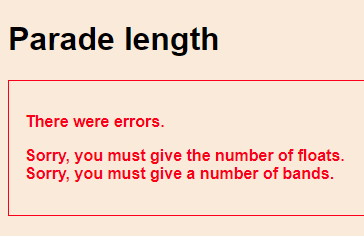Show all messages that apply.

Here's some output, for `?floats=3&amp;bands=2`: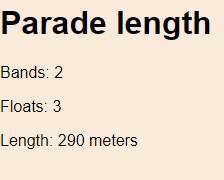You can try my solution.

Submit your URL, and a zip of your files.

### What now?

We're starting to get a lot of code. Let's organize it a bit.